# Material for 3rd Year Engineering Materials (Polymers)

## Solutions

Following some students' enquiries, here are the solutions and marking scheme to the Summer 2004 exam paper [PDF, 412kb]. The marking scheme for question 5 is not marked on the PDF, but it is pretty straight forward.
1. Maxwell 
2. Kelvin 
3. Standard-Linear-Solid plus Discussion 
4. Numerical calculation 
To give a total of 20 marks for the question

### A note on Question 5

When answering this question, some people misinterpreted the expression for creep compliance in Question 5, and interpreted one or both of the minus signs as multiplications.The misinterpretations gave rise to a less-realistic viscoelastic model, and it is important to see why. If we interpret it correctly, then at t=0, the value of D begins as (omitting units) 2-exp(0)=2-1=1. As t increases, the exponential term decreases from one towards a limit of zero. This means that D gradually increases from 1.0 towards a limit of 2.0-0.0=2.0

This is plotted in the figure shown (click for a full-size view), where the red curve shows the correct interpretation. Also plotted are other ways that D was interpreted. By comparing these with the behaviour discussed in the first parts of Question 5, it is easy to see that these alternative models are not at all realistic.

## Handouts

### Lectures

1. Lecture 1 -- Introduction (no handout available)
2. Lecture 2 -- Polymerisation reactions
3. Lecture 3 -- RMM, MWD, Material properties, Crystallisation, Amorphous/Crystalline structures, Glass transition temperature
Acetates: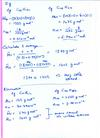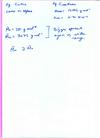Or all in one file
4. Lecture 4 -- Introduction to Viscoelasticity Acetate:5. Lecture 5 -- Viscoelasticity (no handout available online, short lecture)
Acetates: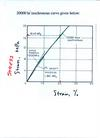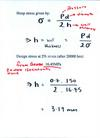Or all in one file
6. Lecture 6 -- Maxwell model, Kelvin model and Standard Linear Solid
Acetates: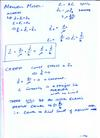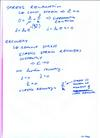Or all in one file
7. Lecture 7 -- Viscoelasticity, Boltzmann Superposition
Acetates: (note that the second worked problem is Q.6 from Summer 1999)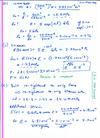Or all in one file